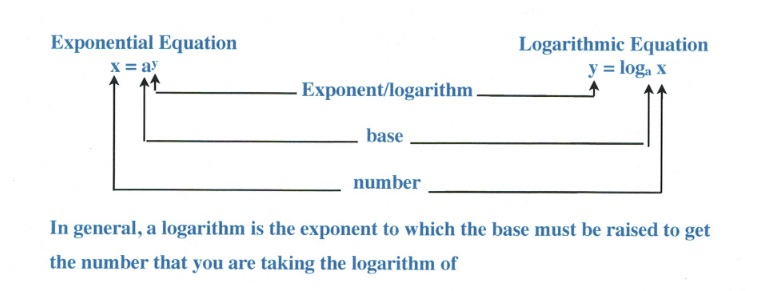Chapter 11: Functions

# 11.5 Logarithmic Functions

Logarithms come from a rich history, extending from the Babylonians around 1500–2000 BC, through the Indian mathematician Virasena around 700–800 AD, and later rapidly growing and expanding in European science from the mid-1500s and on. Logarithms were developed to reduce multiplication and division to correspond to adding and subtracting numbers on a number line. Quite simply, logarithms reduced the complexity of these functions and retained significance until the advent of the computer. Even so, logarithms are still in use today in many functions. This topic is taught here, since the logarithmic function is the inverse to the exponential function (shown in 11.4). We will use this feature to solve both exponential and logarithmic functions.In general, a logarithm is the exponent to which the base must be raised to get the number that you are taking the logarithm of. Using logarithms and exponents together, we can start to identify useful relations.

Consider 23. 23 = 2 × 2 × 2 = 8, when written using logarithmic functions, will look like $\log_{2} 8 = 3$. You read this as the log base 2 of 8 equals 3. This means that, if you are using the base 2 and are looking to find the exponent that yields 8, the power needed on the base is 3. You can quantify this relation in either the one of the two equations: $x = a^y$ or $y = \log_{a} x$. Writing this relationship in either form is illustrated in the following examples.

Example 11.5.1

Write the logarithmic equation for each given exponential relation.

1. $x^3 = 12\hspace{0.5in} \text{In a logarithmic equation looks like}\hspace{0.5in} \log_{x}12 = 3$
2. $4^3 = 64\hspace{0.5in}\text{In a logarithmic equation looks like}\hspace{0.5in} \log_{4}64 = 3$
3. $7^2 = 49\hspace{0.5in}\text{In a logarithmic equation looks like}\hspace{0.5in} \log_{7}49 = 2$
4. $y^6 = 102\hspace{0.5in}\text{In a logarithmic equation looks like}\hspace{0.5in} \log_{y}102 = 6$

Example 11.5.2

Write the exponential relation for each given logarithmic equation.

1. $\log_{x}42=5 \hspace{0.54in} \text{In exponential form looks like }\hspace{0.5in}x^5=42$
2. $\log_{4}624 = 5 \hspace{0.5in}\text{In exponential form looks like }\hspace{0.5in}4^5 = 624$
3. $\log_{3}18 = 2\hspace{0.54in}\text{In exponential form looks like }\hspace{0.5in}3^2 = 18$
4. $\log_{y}12=4\hspace{0.54in}\text{In exponential form looks like }\hspace{0.5in}y^4=12$

A further illustration of this relationship is shown below for the exponents and logarithms for the common base values of 2 and 10.

## Examples of Exponents and Logarithms for Base 2 and 10

$\begin{array}{llll} 2^0=1&\hspace{0.5in}\log_{2}1=0&\hspace{0.5in}10^0=1&\hspace{0.5in}\log_{10}1=0 \\ 2^1=2&\hspace{0.5in}\log_{2}2=1&\hspace{0.5in}10^1=10&\hspace{0.5in}\log_{10}10=1 \\ 2^2=4&\hspace{0.5in}\log_{2}4=2&\hspace{0.5in}10^2=100&\hspace{0.5in}\log_{10}100=2 \\ 2^3=8&\hspace{0.5in}\log_{2}8=3&\hspace{0.5in}10^3=1000&\hspace{0.5in}\log_{10}1000=3 \\ 2^4=16&\hspace{0.5in}\log_{2}16=4&\hspace{0.5in}10^4=10000&\hspace{0.5in}\log_{10}10000=4 \\ 2^5=32&\hspace{0.5in}\log_{2}32=5&\hspace{0.5in}10^5=100000&\hspace{0.5in}\log_{10}100000=5 \\ 2^6=64&\hspace{0.5in}\log_{2}64=6&\hspace{0.5in}10^6=1000000&\hspace{0.5in}\log_{10}1000000=6 \\ 2^7=128&\hspace{0.5in}\log_{2}128=7&\hspace{0.5in}10^7=10000000&\hspace{0.5in}\log_{10}10000000=7 \\ 2^8=256&\hspace{0.5in}\log_{2}256=8&\hspace{0.5in}10^8=100000000&\hspace{0.5in}\log_{10}100000000=8 \end{array}$

In the following examples, we evaluate logarithmic functions by converting the logarithms to exponential form.

Example 11.5.3

Evaluate the logarithmic equation $\log_{2}64 = x$.

The exponential form of this logarithm is $2^x = 64$.

Since 64 equals 26, rewrite this as $2^x = 2^6$, which means that $x = 6$.

Often, you are asked to evaluate a logarithm that is not in the form of an equation; rather, it is given as a simple logarithm. For this type of question, set the logarithm to equal $x$ and then solve as we did above.

Example 11.5.4

Evaluate the logarithmic equation $\log_{125}5$.

First, we set this logarithm to equal $x$, so $\log_{125}5 = x$.

The exponential form of this logarithm is $5^x = 125$.

Since 125 equals 53, we rewrite this as $5^x = 5^3$, which means that $x = 3$.

Logarithmic equations that appear more complicated are solved using a somewhat similar strategy as above, except that you often employ algebraic methods. For instance:

Example 11.5.5

Evaluate the logarithmic equation $\log_{2}(3x + 5) = 4$.

The exponential form of this logarithm is $2^4 = 3x + 4$.

This now becomes an algebraic equation to solve:

$\begin{array}{rrrrr} 16&=&3x&+&4 \\ -4&&&-&4 \\ \hline \dfrac{12}{3}&=&\dfrac{3x}{3}&& \\ \\ x&=&4&& \end{array}$

The most common form of logarithm uses base 10. This can be compared to the most common radical of the square root. When encountering base 10 logarithms, they are often written without the base 10 shown. To solve these, rewrite in exponential form using the base 10.

Example 11.5.6

Evaluate the logarithmic equation $\log x = -2$.

The exponential form of this logarithm is $10^{-2} = x$.

Since $10^{-2} = \dfrac{1}{100}$, this means that $x = \dfrac{1}{100}$.

# Questions

Rewrite each equation in exponential form.

1. $\log_{9}81=2$
2. $\log_{b}a=-16$
3. $\log_{7}\dfrac{1}{49}=-2$
4. $\log_{16}256=2$
5. $\log_{13}169=2$
6. $\log_{11}1=0$

Rewrite each equation in logarithmic form.

1. $8^0=1$
2. $17^{-2}=\dfrac{1}{289}$
3. $15^2=225$
4. $144^{\frac{1}{2}}=12$
5. $64^{\frac{1}{6}}=2$
6. $19^2=361$

Evaluate each expression.

1. $\log_{125}5$
2. $\log_{5}125$
3. $\log_{343}\dfrac{1}{7}$
4. $\log_{7}1$
5. $\log_{4}16$
6. $\log_{4}\dfrac{1}{64}$
7. $\log_{6}36$
8. $\log_{36}6$
9. $\log_{2}64$
10. $\log_{3}243$

Solve each equation.

1. $\log_{5}x=1$
2. $\log_{8}k=3$
3. $\log_{2}x=-2$
4. $\log n=3$
5. $\log_{11}k=2$
6. $\log_{4}p=4$
7. $\log_{9}(n+9)=4$
8. $\log_{11}(x-4)=-1$
9. $\log_{5}(-3m)=3$
10. $\log_{2}-8r=1$
11. $\log_{11}(x+5)=-1$
12. $\log_{7}-3n=4$
13. $\log_{4}(6b+4)=0$
14. $\log_{11}(10v+1)=-1$
15. $\log_{5}(-10x+4)=4$
16. $\log_{9}(7-6x)=-2$
17. $\log_{2}(10-5a)=3$
18. $\log_{8}(3k-1)=1$